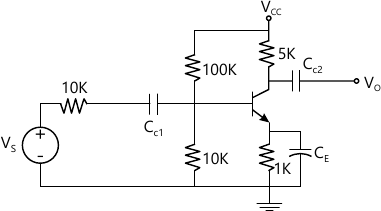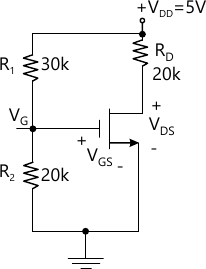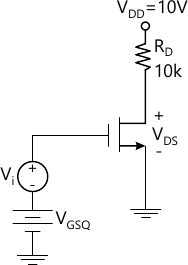MORE IN Electronic Devices & Circuits
SPPU Electronics and Telecom Engineering (Semester 3)
Electronic Devices & Circuits
June 2015
Total marks: --
Total time: --
INSTRUCTIONS
(1) Assume appropriate data and state your reasons
(2) Marks are given to the right of every question
(3) Draw neat diagrams wherever necessary

Answer any one question from Q1 and Q2
1 (a) List the sources of instability of collector current. Explain self-bias circuit in detail.
6 M
1 (b) The transistor in the given circuit is connected as a common emitter amplifier. Calculate Av, Ri, Ro, Refer Fig 1. Assume hie=1.1 KΩ, hfe=50, hre=2.5 ×10-4, hoe=1/40 k.6 M

2 (a) Write a short note on thermal runaway. Explain thermal Stability.
6 M
2 (b) Describe the method to increase the input resistance using Darlington connection.
6 M

Answer any one question from Q3 and Q4
3 (a) Draw and explain hybrid- common emitter transistor Model.
6 M
3 (b) In Colpitt's oscillators L2 = 5 μH, C1 = C2 = 0.001 F. What will be the frequency of oscillations. If value of inductor is doubled what will be frequency of oscillations ? What should be the value of inductor to get frequency double to that of original frequency ?
6 M

4 (a) For three stage RC coupled amplifier overall upper 3 dB frequency is 16 kHz and overall lower 3 dB frequency is 25 Hz. What are the values of F L and F H for each stage ? Assume all stages identical. Also calculate bandwidth of each stage.
6 M
4 (b) Draw and explain various topologies of negative feedback.
6 M

Answer any one question from Q5 and Q6
5 (a) What is cross over distortion ? Describe a method to minimize this distortion.
6 M
5 (b) A class-A amplifier operates from VCC=20 V, draws a no signal current of 5 Amp and feeds a load of 40 Ω, through a step up transformer of N2/N1 = 3.16 Find:
i) Whether the amplifier is properly matched for maximum power transfer?
ii) Maximum a.c. signal power output
iii) Maximum d.c. power input
iv) Conversation efficiency at maximum at maximum signal input.
7 M

6 (a) Draw and explain complementary symmetry class-B power amplifier.
6 M
6 (b) A power amplifier supplies 3 watt to a load of 6 k . The zero signal d.c. collector current is 55 mA and the collector current with signal is 60 mA. How much is the percentage second harmonic distortion.
6 M

Answer any one question from Q7 and Q8
7 (a) Explain the following non-ideal current voltage characteristics of MOSFET:
(i) Body effect
(ii) Temperature effects
(iii) Breakdown effects.
6 M
7 (b) Calculate the drain current and drain to source voltage of a common source circuit with an N-channel EMOSFET shown in Fig. 2. Find the power dissipated in the transistor. Given VTN=1 V and Kn=0.1 mA/V2.7 M

8 (a) Determine the small signal voltage gain for CS amplifier shown in Fig 3. Transistor parameters are VTN=2 V, Kn=0.5 mA/V2 and λ=0. Assume the transistor is biased such that IDQ=0.4 mA.7 M
8 (b) Write a short note on Bi-CMOS technology.
6 M

More question papers from Electronic Devices & Circuits